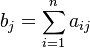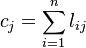## Definition

Backward Linkage captures the interconnection of an industry to other industries from which it purchases its inputs in order to produce its output.

In the simplest forms linkages are measured as summations of the appropriate IO matrix

The proportion of intermediate consumption to the total output of the sector. In terms of the Technical Coefficient Matrix this is expressed as the sum$b_j = \sum_{i=1}^{n} a_{ij}$

Expressed in terms of transactions (Z, Input-Output Matrix not A) it captures the value of total intermediate inputs for a sector j as a proportion of the value of j’s total output.$c_j = \sum_{i=1}^{n} l_{ij}$